# Precision Resolver-to-Digital Converter Measures Angular Position and Velocity

### Introduction

Resolvers, electromechanical sensors that measure precise angular position, operate as variable coupling transformers, with the amount of magnetic coupling between the primary winding and two secondary windings varying according to the position of the rotating element (rotor), which is typically mounted on the motor shaft. Employed in industrial motor controls, servos, robotics, power-train units in hybrid- and full-electric vehicles, and many other applications that require precise shaft rotation, resolvers can withstand severe conditions for a very long time, making them the perfect choice for military systems in harsh environments.

Standard resolvers have a primary winding on the rotor and two secondary windings on the stator. Variable reluctance resolvers, on the other hand, have no windings on the rotor. Their primary and secondary windings are all on the stator, but the saliency (exposed poles) of the rotor couples the sinusoidal variation in the secondary with the angular position. Figure 1 shows classical and variable reluctance resolvers.

When the primary winding, R1R2, is excited with a sinusoidal signal as expressed in Equation 1, a signal is induced in the secondary windings. The amount of coupling onto the secondary windings is a function of the position of the rotor relative to that of the stator, and an attenuation factor known as the resolver transformation ratio. Because the secondary windings are displaced mechanically by 90°, the two output sinusoidal signals are phase shifted by 90° with respect to each other. The relationships between the resolver input and output voltages are shown in Equation 2 and Equation 3. Equation 2 is the sine signal; Equation 3 is the cosine signal.(1)(2)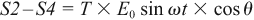(3)

where: θ is the shaft angle, ω is the excitation signal frequency, E0 is the excitation signal amplitude, and T is the resolver transformation ratio.

The two output signals are modulated by the sine and cosine of the shaft angle. A graphical representation of the excitation signal and the sine and cosine output signals is shown in Figure 2. The sine signal has maximum amplitude at 90° and 270° and the cosine signal has maximum amplitude at 0° and 180°.

A resolver sensor has a unique set of parameters that should be considered during the design phase. The most critical electrical parameters and the respective typical specifications are summarized in Table 1.

Table 1. Resolver Key Parameters

 Electrical Parameter Typical Range Unit Description Input Voltage 3–7 V rms Recommended excitation signal amplitude to be applied to resolver primary winding R1 – R2 Input Frequency 50–20,000 Hz Recommended excitation signal frequency to be applied to resolver primary winding R1 – R2 Transformation Ratio 0.2–1.0 V/V Ratio between the primary and secondary windings signal amplitude Input Impedance 100–500 Ω Input impedance of resolver Phase Shift ±25 degrees Phase shift between excitation signal applied to primary winding (R1 – R2) and sine/cosine signals on secondary windings (S3 – S1, S2 – S4) Pole Pairs 1–3 Number of electrical rotations per mechanical rotation

### Resolver-to-Digital Converter

The primary winding is excited with the sine wave reference signal, and two differential output signals, sine and cosine, are electromagnetically induced on the secondary windings. Interfacing between the resolver and the system microprocessor, a resolver-to-digital converter (RDC) uses these sine and cosine signals to decode the angular position and rotation speed of the motor shaft.

A majority of RDCs uses a Type-II tracking loop to perform position and velocity calculations. Type-II loops use a second-order filter to ensure that steady-state errors are zero for stationary or constant-velocity input signals. The RDC simultaneously samples both input signals to provide digitized data to the tracking loop. The newest example of an RDC that uses this type of loop is ADI’s AD2S1210 complete 10-bit to 16-bit tracking converter, whose on-chip programmable sinusoidal oscillator provides the excitation signal for the primary winding.

As specified in Table 1, a typical resolver requires a low-impedance 3-V rms to 7-V rms signal to drive the primary winding. Operating on a 5-V supply, the RDC typically delivers a 7.2-V p-p differential signal on the excitation outputs. This signal does not have sufficient amplitude and drive capability to meet the resolver’s input specifications. In addition, resolvers attenuate signals by up to 5×, so the resolver output amplitude does not meet the RDC’s input amplitude requirements, shown in Table 2.

A solution to this problem is to use a differential amplifier to boost the sinusoidal signal to the primary. This amplifier must be able to drive loads as low as 100 Ω. A common practice is to drive the primary with a large signal to obtain a good signal-to-noise ratio. The output sine and cosine signals can then be attenuated with a resistor divider.

In many industrial and automotive applications, RDCs are used in noisy environments, which can induce high-frequency noise into the sine and cosine lines. To solve this, insert a simple differential low-pass filter as close as possible to the RDC. Figure 3 shows a typical resolver-to-digital converter interface including amplifier and filter.

### Theory of Operation

Figure 4 shows the operational blocks in the RDC. The converter continuously tracks the shaft angle θ by producing an output angle ϕ, which is fed back and compared to the input angle. The resulting error between the two angles is minimized when the converter is tracking the position.

To measure the error, multiply the sine and cosine inputs by cos(ϕ) and sin(ϕ) respectively:(4)(5)

Next, take the difference between the two:(6)

Then, demodulate the signal using the internally generated synthetic reference:(7)

Using a trigonometric identity, E0 (sin θ cos ϕ – cos θ sin ϕ) = E0 sin (θϕ), which is approximately equal to E0 (θϕ) for small values of angular error (θϕ). E0 (θϕ) is the difference between the angular error of the rotor and the digital angle output of the converter. The Type-II tracking loop operates to null the error signal. When this is accomplished, ϕ equals the resolver angle θ.

### Key RDC Parameters

Engineers must consider a number of parameters used to characterize resolver-to-digital converters before selecting an appropriate device. Table 2 shows key RDC parameters and specifications for the AD2S1210, which sets the boundaries as the best-in-class converter.

Table 2. Key RDC Parameters and Values for the AD2S1210
 Electrical Parameter Typical Range Unit Description Input Voltage 2.3–4.0 V p-p Differential signal range for the sine and cosine inputs Phase Lock Range ±44 degrees Phase shift between the excitation signal generated by the RDC and the sine and cosine inputs Angular Accuracy ±2.5 arc min Angular accuracy of the RDC Resolution 10, 12, 14, 16 bits RDC resolution Velocity Accuracy 2 LSB Velocity precision offered by the RDC Tracking Rate 3125, 1250, 625, 156 rps Tracking capabilities at specific resolutions Settling Time 2.2, 6, 14.7, 66 ms Converter response time to a 179° step change at specific resolutions

### Error Sources

The accuracy of a complete system is determined by the accuracy of the RDC, as well as errors from the resolver, system architecture, cabling, excitation buffer, and sine/cosine input circuitry. The most common sources of system error are amplitude mismatch, signal phase shift, offsets, and acceleration.

Amplitude mismatch is the difference in the peak-to-peak amplitudes of the sine and cosine signals when they are at their peak amplitudes, 0° and 180° for cosine, 90° and 270° for sine. Mismatch can be introduced by variation in the resolver windings, or by the gain between the resolver and the RDC’s sine and cosine inputs. Equation 3 can be rewritten as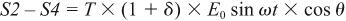(8)

where δ is the percentage amplitude mismatch of the cosine signal relative to the sine signal. The static position error, ε, expressed in radians, is defined as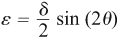(9)

Equation 9 shows that the amplitude mismatch error oscillates at twice the rate of rotation, with a maximum of δ/2 at odd integer multiples of 45°, and no error at 0°, 90°, 180°, and 270°. With a 12-bit RDC, 0.3% amplitude mismatch will result in approximately 1 LSB of error.

The RDC accepts differential sine and cosine signals from the resolver. The resolver removes any dc component from the carrier, so a VREF/2 dc bias must be added to ensure that the resolver output signals are in the correct operating range for the RDC. Any offset in the dc bias between SIN and SINLO inputs or COS and COSLO inputs introduces an additional system error.

The error introduced by the common-mode offset is worse in the quadrants where the sine and cosine signal carriers are in antiphase to each other. This occurs for positions between 90° and 180° and 270° and 360°, as shown in Figure 5. Common-mode voltages between the terminals offset the differential signal by twice the common-mode voltage. The RDC is ratiometric, so perceived changes in amplitude of the incoming signals cause an error in position.

Figure 6 shows that even when the differential peak-to-peak amplitude of the sine and cosine are equal, the perceived amplitudes of the incoming signals are different. The worst case error will occur at 135° and 315°. At 135°, A = B in an ideal system, but A ≠ B in the presence of offset, so a perceived amplitude mismatch occurs.

Another source of error is differential phase shift, which is the phase shift between the resolver’s sine and cosine signals. Some differential phase shift will be present on all resolvers as a result of coupling. A small resolver residual voltage or quadrature voltage indicates a small differential phase shift. Additional phase shift can be introduced if the sine and cosine signal lines have unequal cable lengths or drive different loads.

The differential phase of the cosine signal relative to the sine signal is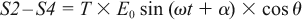(10)

where α is the differential phase shift.

Solving for the error introduced by α yields the error term, ε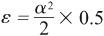(11)

where α and ε are expressed in radians.

Most resolvers also introduce a phase shift between the excitation reference signal and the sine and cosine signals, causing an additional error, ε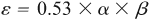(12)

where β is the phase shift between the sine/cosine signals and the excitation reference signal.

This error can be minimized by choosing a resolver with a small residual voltage, ensuring that the sine and cosine signals are handled identically, and by removing the reference phase shift.

Under static operating conditions, phase shift between the excitation reference and the signal lines will not affect the converter’s accuracy, but resolvers at speed generate speed voltages due to the reactive components of the rotor impedance and the signals of interest. Speed voltages, which only occur at speed, not at static angles, are in quadrature to the signal of interest. Their maximum amplitude is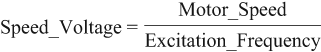(13)

In practical resolvers, rotor windings include both reactive and resistive components. The resistive component produces a nonzero phase shift in the reference excitation that is present when the rotor is both at speed and static. Together with the speed voltages, the nonzero phase shift of the excitation produces a tracking error that can be approximated as(14)

To compensate for the phase error between the resolver reference excitation and sine/cosine signals, the AD2S1210 uses the internally filtered sine and cosine signals to synthesize an internal reference signal in phase with the reference frequency carrier. Generated by determining the zero crossing of either the sine or cosine (whichever is larger, to improve phase accuracy) and evaluating the phase of the resolver reference excitation, it reduces the phase shift between the reference and sine/cosine inputs to less than 10°, and operates for phase shifts of ±44°. A block diagram of the synthetic reference block is shown in Figure 7.

The advantage of Type-II tracking loops over Type-I loops is that no positional error occurs with constant velocity. Even in a perfectly balanced system, however, acceleration will create an error term. The amount of error due to acceleration is determined by the control-loop response. Figure 8 shows the loop response for the AD2S1210.

The loop acceleration constant, Ka, is(15)

where the loop coefficients change depending on the resolution, input signal amplitude, and the sampling period. The AD2S1210 samples twice during each CLKIN period.

Table 3. RDC System Response Parameters
 Parameter Description 10-Bit Resolution 12-Bit Resolution 14-Bit Resolution 16-Bit Resolution k1 ADC gain Input voltage/ref voltage = (3.15/2)/2.47 (nominal) k2 Error gain 12π × 106 36π × 106 164π × 106 132π × 106 a Compensator  zero coefficient 8187/8192 4095/4096 8191/8192 32,767/32,768 b Compensator  pole coefficient 509/512 4085/4096 16,359/16,384 32,757/32,768 c Integrator gain 1/220 1/222 1/224 1/226 T Sampling period 1/(CLKIN/2)

The tracking error due to acceleration can then be calculated as(16)

Figure 9 shows the angular error due vs. acceleration for different resolution settings.

### Input Filter

For best system accuracy, connect the resolver outputs directly to the AD2S1210 SIN, COS, SINLO, and COSLO pins to reduce mismatch or phase shifts. This is not always feasible, however. It may be necessary to attenuate the resolver’s sine and cosine signals to match the RDC’s input specifications, some signal filtering may be required due to the noisy environment, and ESD or short circuit protection may be required at the resolver connector.

Figure 10 shows a typical interface circuit between the resolver and the AD2S1210. The series resistors and the diodes provide adequate protection to reduce the energy of external events such as ESD or shorts to supply or ground. These resistors and the capacitor implement a low-pass filter that reduces high-frequency noise that couples onto the resolver inputs as a result of driving the motor. It may also be necessary to attenuate the resolver sine and cosine input signals to align with the RDC’s input voltage specification. This can be accomplished by the addition of resistor RA. The AD2S1210 has internal circuitry to bias the SIN, SINLO, COS, and COSLO to VREF/2. This weak bias can be easily overdriven. A simple method to achieve this is the inclusion of 47-kΩ resistors RB, which will bias the signals to 2.5 V.

### Excitation Buffer

A buffer is typically required to drive the resolver’s low-impendence inputs. This excitation buffer can be implemented in many ways, two of which are shown here. The first circuit is commonly used in automotive and industrial designs, while the second simplifies design by replacing the standard push-pull architecture with a high output current amplifier.

The high-current driver shown in Figure 11 amplifies and level shifts the reference oscillator output. The driver uses an AD8662 dual, low-noise, precision op amp and a discrete emitter follower output stage. A duplicate buffer circuit provides a fully differential signal to drive the resolver’s primary winding.

This high-current buffer offers drive capability, gain range, and bandwidth optimized for a standard resolver, and it can be adjusted to meet specific requirements of the application and sensor, but the complex design has a number of disadvantages in terms of component count, PCB size, cost, and engineering time needed to alter it to application-specific needs.

The design can be optimized by replacing the AD8662 with an amplifier that provides the high output current required for driving resolvers directly, simplifying the design and eliminating the need for a push-pull stage.

The high-current driver shown in Figure 12 uses the AD8397 high-current dual op amp with rail-to-rail outputs to amplify and level shift the reference oscillator output, optimizing the interface to the resolver. The AD8397 achieves low-distortion, high output current, and wide dynamic range, making it ideal for use with resolvers. With 310-mA current capability for 32-Ω loads, it can deliver the required power to a resolver without the use of the conventional push-pull stage, simplifying the driver circuit and reducing power consumption. A duplicate circuit provides a fully differential signal to drive the primary winding. Available in an 8-lead SOIC package, the AD8397 is specified over the –40°C to +85°C extended industrial temperature range.

The passive component values can be altered to change the output amplitude and common-mode voltage, with the output amplitude set by the amplifier gain, R2/R1, and the common-mode voltage set by R3 and R4.

Capacitor C1 and resistor R2 form a low-pass filter to minimize noise on the EXC and EXC outputs. The capacitor should be chosen to minimize phase shift of the carrier. The total phase shift between the excitation output and the sine and cosine inputs should not exceed the phase-lock range of the RDC. The capacitor is optional, as classical resolvers filter out high-frequency components exceptionally well.

Figure 13 shows the AD8397 reference buffer compared with a traditional push-pull circuit. An FFT analyzer measured power of the fundamental and harmonics on the excitation signals of the AD2S1210.

The power of each fundamental shows little discrepancy between both configurations, but the AD8397 buffer has reduced harmonics. Although the AD8397 circuit offers slightly lower distortion, both buffers provide adequate performance. Eliminating the push-pull stage simplifies the design, uses less space, and consumes less power as compared to a conventional circuit.

### Conclusion

When combined with the AD2S1210 resolver-to-digital converter, resolvers can create a high-precision, robust control system for position and velocity measurements in motor-control applications. To achieve the best overall performance, buffer circuits based on the AD8662 or the AD8397 are required to amplify the excitation signals and provide the drive strength required by the resolver. To complete the system, a basic input circuit can provide signal conditioning as required. As is the case with all mixed-signal mechatronic signal chains, care must be taken to design an accurate system that considers all error sources. With its variable resolution, reference generation, and on-chip diagnostics, the AD2S1210 provides an ideal RDC solution for resolver applications. It is available in industrial and automotive grades.

### References

High Current Driver for the AD2S1210 Resolver-to-Digital Reference Signal Output

Circuit Note CN-0276. High Performance, 10-Bit to 16-Bit Resolver-to-Digital Converter.

Circuit Note CN-0192. High Current Driver for the AD2S1210 Resolver-to-Digital Reference Signal Output.